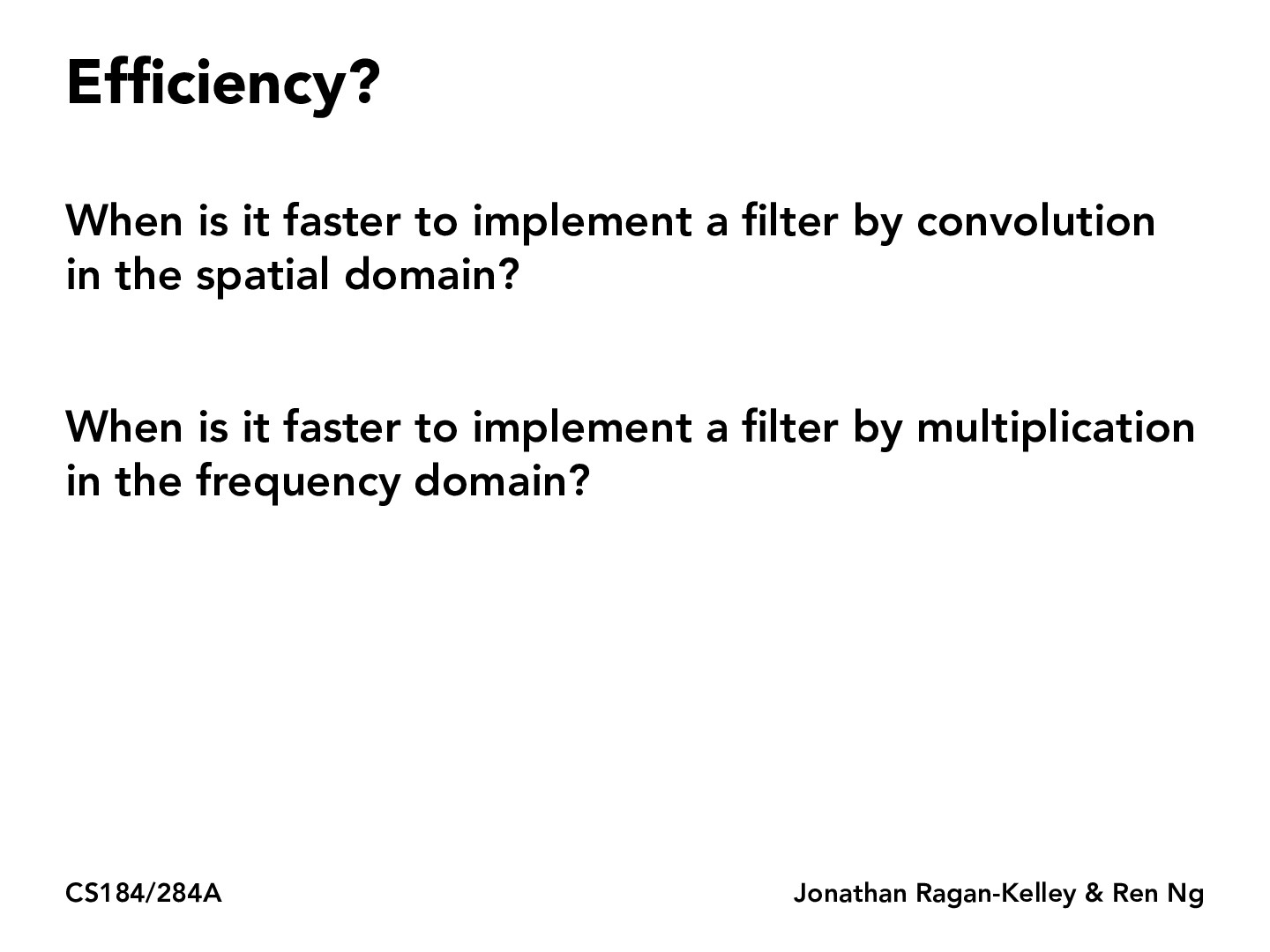Lecture 3: Antialiasing (67)upasanachatterjee

In lecture, JRK says that choosing convolution or FT will depend on the relative sizes of the filters in the domains and the cost of running the FT algorithm. I was wondering if we would ever need to factor in the cost of creating the frequency domain of an image, or if that's always given/cheap to calculate?killawhale2

I assume that the cost would be the cost of taking the FT. If we recall that efficient algorithms for the FT exist such as the FFT, I would assume that the cost of taking things to the frequency domain is pretty low.

You must be enrolled in the course to comment